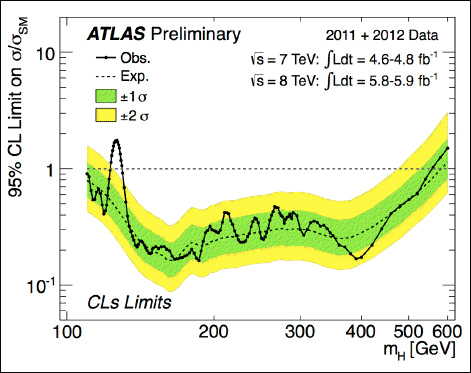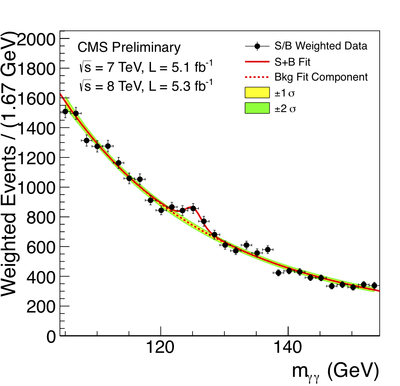## Higgs boson: now more discovered! Or is it?

Well, there you go… the webcast from CERN is over, the results have been announced: CMS and ATLAS have discovered the Higgs boson respectively at $$\SI{125.3 +- 0.6}{GeV}$$ with a statistical significance of $$4.9\sigma$$, and at $$\SI{126.5}{GeV}$$ with a statistical significance of $$5.0\sigma$$!Okay, to be fair, it didn’t take much (any) imagination to predict that result a mere 12 hours before it was announced. The rumors that were flying around over the past day turned out to be basically true. But the LHC detectors actually performed better than I would have thought — whereas I was expecting them to have to combine their results to constitute a discovery by the standard particle physicists use, the two experiments were actually both able to do it individually. (So what if CMS was $$0.1\sigma$$ short — that’s close enough as far as I’m concerned.)
There are actually some tantalizing hints on this already. Both experiments looked for several different decay channels — ways that the Higgs boson could decay into the particles that are actually detected. If this particle really is the standard model Higgs boson, the results from each of these decay channels should individually match up with the predictions. But that’s not true in every case! CMS investigated the decay $$H\to\tau\bar\tau$$ (Higgs boson decaying into a tau lepton and its antiparticle), and they found a conspicuous absence of those events. This could easily be a statistical fluke, because even if the Higgs boson is there, it wouldn’t cause very many $$\tau\bar\tau$$ decays, but it could also be the first signal that the particle discovered is not the boring old standard model Higgs after all! And that would be very exciting. I’ll certainly be looking forward to see what ATLAS comes up with when they do the corresponding analysis on their own data.